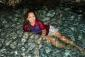# Differential Equation(xy + (y/x) + (3/x) -2) dy + y2 dx = 2(1+(y/x))dx

• Mathematics inside the configured delimiters is rendered by MathJax. The default math delimiters are $$...$$ and $...$ for displayed mathematics, and $...$ and $...$ for in-line mathematics.